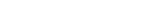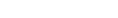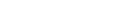# What is the Special Theory of Relativity?

The Special Theory of Relativity is a theory that explains the relationship between space and time. It was invented by Albert Einstein to explain the inconsistency between Newtonian Mechanics and Maxwell’s Equations of Electromagnetism. The theory is based on two postulates: 1) The laws of physics hold in non-accelerating frames of reference and 2) The speed of light in a vacuum remains constant for all observers. The theory explains phenomena such as the relativity of simultaneity and length contraction.

When it comes to the human perception of space and time, we have a rather straightforward approach. Our understanding of space is three-dimensional, while our concept of time is very linear. However, what if I told you that time and space do not behave the same way in all situations when we consider the physics of very high-velocity objects. Basically, the special theory of relativity establishes the relationship between space and time for problems that classical physics (Newtonian physics) fail to understand or explain.

Recommended Video for you:

## The Postulates

The whole premise upon which special relativity is built consists of two simple postulates:

1. The laws of physics hold in non-accelerating frames of reference.
2. The speed of light in a vacuum remains constant for all observers.

The reason these postulates were framed was that Einstein found an inconsistency when he tried to apply Newtonian Mechanics to Maxwell’s equations of electromagnetism. This led Einstein to believe that Newtonian Mechanics did not work according to Maxwell’s Equations of Electromagnetism. However, it was not possible for the speed of time to be a variable, as it had been rigorously analyzed and experimented upon by numerous scientists. Although Newtonian physics were highly instrumental when it came to everyday calculations, they failed miserably when considering high-velocity physics (physics at the speed of light).

## The Mathematical Foundation

The funny thing about the special theory of relativity was that the math required for it had been invented before the theory itself! Albert Einstein went through many mathematical tools before finally settling on the mathematical framework of Hendrik Lorentz. Hendrik Lorentz was a Dutch physicist who invented the Lorentz transformation, which helped accommodate the notion that multiple frames of reference can be calculated simultaneously (the foundation upon which the special theory of relativity was written). Using the infamous equations of Lorentz Transformation, Albert Einstein published the scientific paper “On the electrodynamics of moving bodies”, which we today know as the Special Theory of Relativity. The equations are as follows:## Phenomena Explained by Special Relativity

The case point scenarios where special theory comes into play can be proven through Lorentz transformation. Special cases where the physics calculations must be performed at velocities close to the speed of light are where special relativity comes to the fore.

The first situation we will discuss is the relativity of simultaneity. This is when two events appear to occur at two different locations simultaneously in the reference frame of one observer, but may also appear to happen non-simultaneously in the frame of another observer. To take an example, let’s consider a man standing on a moving platform. There are two light sources equidistant from him. Now, let another man be present at a different frame of reference than the man on the platform. Whether the platform moves relative to the man or the other way around, both observers do not report the same timing of the light sources flashing! The man on the train always reports that both light beams are produced simultaneously, but the man observing from the platform reports one light beam being faster than the other (the one closest to him). How can this be and who is wrong?The observer moving relative to the platformThe platform moving relative to the observer

The answer to this question is that neither observer is wrong. The two observers disagree on the timing of the event and the synchronicity with which the events occur. This is because both observers view the same event from different spatial orientations to the relative motion of the occurring event. Therefore, the same event’s occurrence appears differently for different observers due to the relative motion being observed separately. This is relativity of simultaneity.

The second situation where this theory comes into play is length contraction. This is a very surprising phenomenon arguing that the measurement of an object by one observer might be highly different from that of another observer. The simplest way to explain this is with another “moving platform” example. If you were standing on Earth and a spaceship were to pass above you at 10% the speed of light you would be able to see the spaceship and note its length. Now, if you saw the same spaceship pass you a second time at 85% the speed of light, you would see a significantly shorter spaceship. At 99% the speed of light, you would probably see nothing but a line.One of the final points we will touch on is causality and the prohibition of motion faster than the speed of light. This is by far the most interesting principle that we can deduce from the special theory of relativity. The meaning of causality in classical physics implies that no action can occur before its cause. In Einstein’s theory of special relativity, causality means that an effect cannot occur from a cause that is not in the back (past) light cone of that event.(Photo Credit : K. Aainsqatsi/Wikimedia Commons)

A light cone is the path that a flash of light takes when emanating from a single point in space and traveling in all directions of spacetime. If you imagine the current event (or the present moment) to be a plane horizon, then the light from that spreads out as a growing circle of events, resulting in a cone. This light cone that is formed is known as the future light cone. This light cone is a mathematical representation of all future events that will occur after the event horizon. The inverse of this future light cone is what we call the past light cone. If anything were to cross the speed of light, numerous paradoxes would begin to occur. One famous hypothesis is that if one were to travel faster than the speed of light, there is a high chance that instead of going into the future light cone, one would be able to break the space-time barrier and move into the past light cone. Therefore, most scientists feel that it will be easier to travel into the future than into the past.

There are other phenomena that can be explained with the help the of the special theory of relativity, such as time dilation, the composition of velocities and certain optical effects. It may have taken a while for the public to understand or accept Einstein’s wild postulations, but over time, we have found that his scientific theories – and his genius-level reputation – continue to be supported and validated!

References